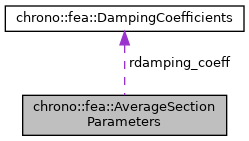chrono::fea::AverageSectionParameters Struct Reference

## Description

The aeverage section properties of tapered section could be stored in this struct.

#include <ChBeamSectionTaperedTimoshenko.h>

Collaboration diagram for chrono::fea::AverageSectionParameters:[legend]

## Public Attributes

double mu
mass per unit length

double alpha

double Cy
elastic center y displacement respect to centerline

double Cz
elastic center z displacement respect to centerline

double Sy
shear center y displacement respect to centerline

double Sz
shear center z displacement respect to centerline

double My
mass center y displacement respect to centerline

double Mz
mass center z displacement respect to centerline

double Jyy
inertia Jyy per unit lenght, in centerline reference, measured along centerline main axes

double Jzz
inertia Jzz per unit lenght, in centerline reference, measured along centerline main axes

double Jyz
inertia Jyz per unit lenght, in centerline reference, measured along centerline main axes

double Jxx
inertia Jxx per unit lenght, in centerline reference, measured along centerline main axes

double Qy
mass moment of area along yy, in centerline reference, measured along centerline main axes

double Qz
mass moment of area along zz, in centerline reference, measured along centerline main axes

double mass_phi

double Jmyy
inertia Jyy per unit lenght, in mass center reference, measured along mass principal axes

double Jmzz
inertia Jzz per unit lenght, in mass center reference, measured along mass principal axes

double Jmyz
inertia Jyz per unit lenght, in mass center reference, measured along mass principal axes

double Jmxx
inertia Jxx per unit lenght, in mass center reference, measured along mass principal axes

double Qmy
mass moment of area along yy, in mass center reference, measured along mass principal axes, which is exactly zero in theory.

double Qmz
mass moment of area along zz, in mass center reference, measured along mass principal axes, which is exactly zero in theory.

double EA
axial rigidity

double GJ
torsion rigidity

double GAyy
shear regidity along yy, evaluated in the elastic center and elastic principal axis. GAyy=ky*G*A

double GAzz
shear regidity along zz, evaluated in the elastic center and elastic principal axis. GAzz=kz*G*A

double EIyy
bending regidity about yy, evaluated in the elastic center and elastic principal axis

double EIzz
bending rigidity about zz, evaluated in the elastic center and elastic principal axis

double GAmyy
shear regidity along yy, evaluated in the mass center and mass principal axis

double GAmzz
shear regidity along zz, evaluated in the mass center and mass principal axis

double EImyy
bending regidity about yy, evaluated in the mass center and mass principal axis

double EImzz
bending rigidity about zz, evaluated in the mass center and mass principal axis

double phimy
shear-deformation parameter, phimy = 12*EImzz/(GAmyy*L*L)

double phimz
shear-deformation parameter, phimz = 12*EImyy/(GAmzz*L*L)

double phiy
shear-deformation parameter, phiy = 12*EIzz/(GAyy*L*L)

double phiz
shear-deformation parameter, phiz = 12*EIyy/(GAzz*L*L)

DampingCoefficients rdamping_coeff
damping parameters

double artificial_factor_for_shear_damping
An artificial factor to modify the damping coefficient in the shear deformation, which may be helpful to improve the numerical stability of Timoshenko beam. More...

## ◆ artificial_factor_for_shear_damping

 double chrono::fea::AverageSectionParameters::artificial_factor_for_shear_damping

An artificial factor to modify the damping coefficient in the shear deformation, which may be helpful to improve the numerical stability of Timoshenko beam.

But you need to take care of the final structural damping ratio of your model after assigning this factor.

The documentation for this struct was generated from the following file:
• /builds/uwsbel/chrono/src/chrono/fea/ChBeamSectionTaperedTimoshenko.h